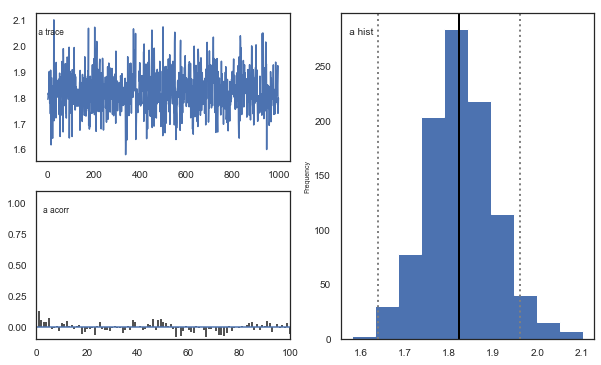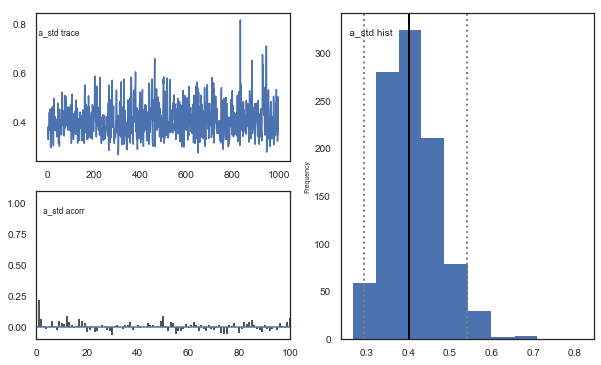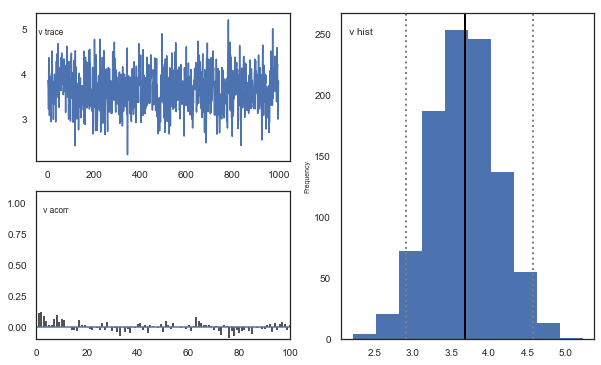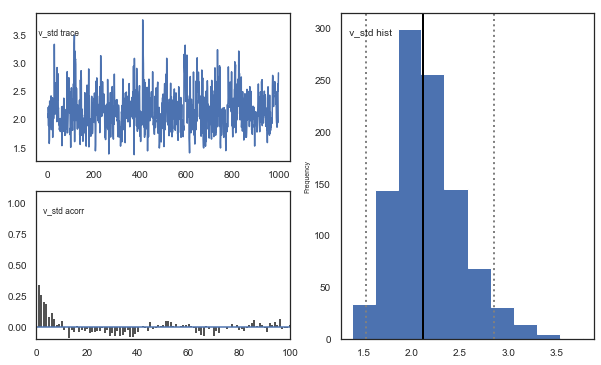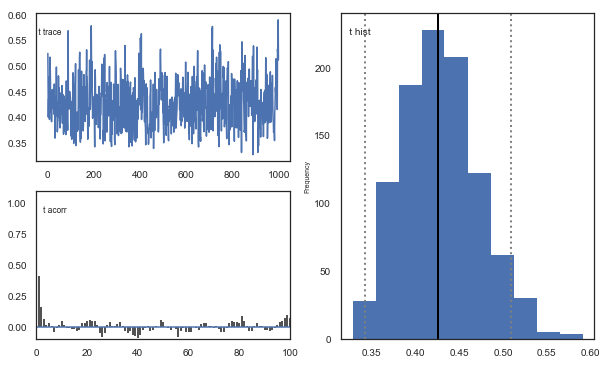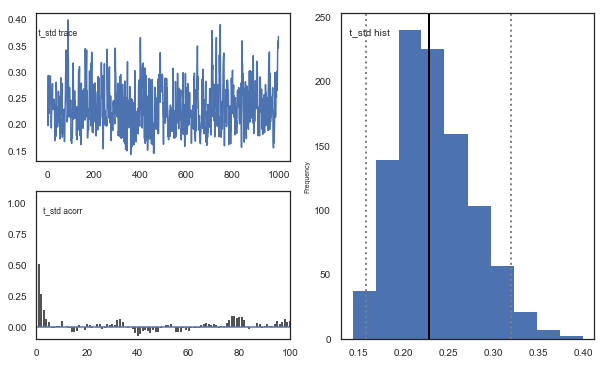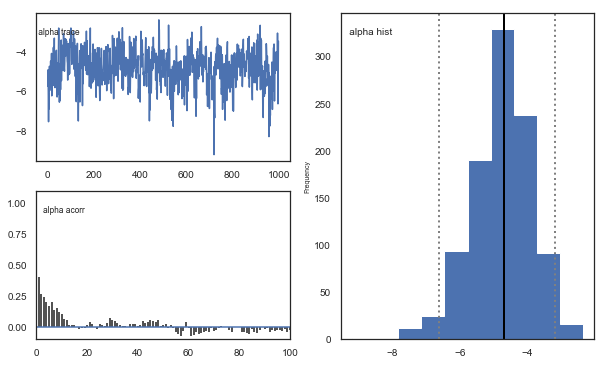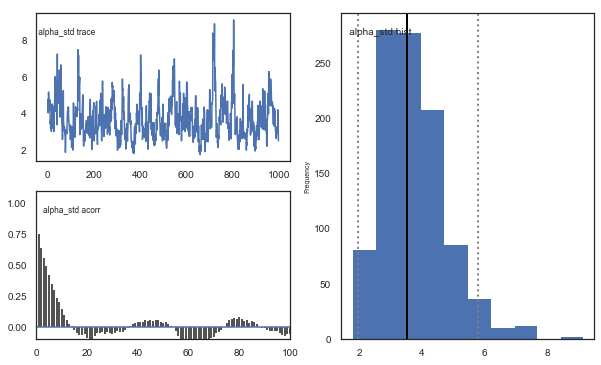# Tutorial for analyzing instrumental learning data with the HDDMrl module¶

This is a tutorial for using the HDDMrl module to simultaneously estimate reinforcement learning parameters and decision parameters within a fully hierarchical bayesian estimation framework, including steps for sampling, assessing convergence, model fit, parameter recovery, and posterior predictive checks (model validation). The module uses the reinforcement learning drift diffusion model (RLDDM), a reinforcement learning model that replaces the standard “softmax” choice function with a drift diffusion process. The softmax and drift diffusion process is equivalent for capturing choice proportions, but the DDM also takes RT distributions into account; options are provided to also only fit RL parameters without RT. The RLDDM estimates trial-by-trial drift rate as a scaled difference in expected rewards (expected reward for upper bound alternative minus expected reward for lower bound alternative). Expected rewards are updated with a delta learning rule using either a single learning rate or with separate learning rates for positive and negative prediction errors. The model also includes the standard DDM-parameters. The RLDDM is described in detail in Pedersen, Frank & Biele (2017). (Note this approach differs from Frank et al (2015) who used HDDM to fit instrumental learning but did not allow for simultaneous estimation of learning parameters).

## 1. Background¶

Traditional RL models typically assume static decision processes (e.g. softmax), and the DDM typically assumes static decision variables (stimuli are modeled with the same drift rate across trials). The RLDDM combines dynamic decision variables from RL and dynamic choice process from DDM by assuming trial-by-trial drift rate that depends on the difference in expected rewards, which are updated on each trial by a rate of the prediction error dependent on the learning rate. The potential benefit of the RLDDM is thus to gain a better insight into decision processes in instrumental learning by also accounting for speed of decision making.

## 2. Installing the module¶

The new version of HDDM (version 0.7.1) that includes the RLDDM is currently not uploaded to conda. So to install you would either have to use pip:
'pip install hddm'
OR
docker: 'pull madslupe/hddm', which runs hddm in jupyter notebook

## 3. How the RLDDM works¶

The main idea of the RLDDM is that reward-based choices can be captured by an accumulation-to-bound process where drift rate is proportional to the difference in expected reward between options, and where expected rewards subsequently are updated in a trial-by-trial basis via reinforcement learning.

drift rate on each trial depends on difference in expected rewards for the two alternatives (q_up and q_low):
drift rate = (q_up - q_low) scaling

where the scaling parameter describes the weight to put on the difference in q-values.

expeceted reward (q) for chosen option is updated according to delta learning rule :
q_chosen = q_chosen + alpha
(feedback-q_chosen)

where alpha weights the rate of learning on each trial.

So in principle all you need is the Wiener first passage time likelihood-function. The reason why HDDM is useful (and hence also HDDMrl) is that it automates the process of setting up and running your model, which tends to be very time consuming. So after structuring the data it is simple to run a model with HDDMrl. In particular it separates subjects and conditions (using the split_by-column, see next section) so that the updating process works correctly, which can be especially difficult to do for RL models.

## 4. Structuring data¶

The HDDMrl module was created to make it easier to model instrumental learning data with the RLDDM. If you are familiar with using HDDM it shouldn't be a big step to start using HDDMrl. Please refresh your memory by starting with the tutorial for HDDM first (especially critical if you have not used HDDM at all). Running HDDMrl does require a few extra steps compared to HDDM, and because the model includes increased potential for parameter colinearity (typically learning rate and the scaling parameter on drift rate) it is even more important to assess model fit, which will be covered below. Here are the most important steps for structuring your dataframe:

1. Sort trials in ascending order within subject and condition, to ensure proper updating of expected rewards.
2. Include a column called 'split_by' which identifies the different task conditions (as integers), to ensure reward updating will work properly for each condition without mixing values learned from one trial type to another.
3. Code the response column with [stimulus-coding] (http://ski.clps.brown.edu/hddm_docs/howto.html#code-subject-responses). Although stimulus-coding and accuracy-coding often are the same in instrumental learning it is important that the upper and lower boundaries are represented by the same alternative within a condition, because expected rewards are linked to the thresholds/boundaries.
4. feedback-column. This should be the reward received for the chosen option on each trial.
5. q_init. Adjusting initial q-values is something that can improve model fit quite a bit. To allow the user to set their own initial values we therefore require that the dataframe includes a column called q_init. The function will set all initial q-values according to the first value in q_init. So this is not the most elegant method of allowing users to set inital value for expected rewards, but it works for now.

#### Required columns in data:¶

• rt: in seconds, same as in HDDM
• response: 0 or 1. defines chosen stimulus, not accuracy.
• split_by: needs to be an integer. Split_by defines conditions (trial-types) that should have the same variables (e.g. Q values) within subject: the trials need to be split by condition to ensure proper updating of expected rewards that do not bleed over into other trial-types. (e.g. if you have stimulus A and get reward you want that updated value to impact choice only for the next stimulus A trial but not necessarily the immediate trial afterwards, which may be of a different condition)
• subj_idx: same as in HDDM, but even more important here because it is used to split trials
• feedback: feedback on the current trial. can be any value.
• q_init: used to initialize expected rewards. can be any value, but an unbiased initial value should be somewhere between the minimum and and maximum reward values (e.g. 0.5 for tasks with rewards of 0 and 1).

## 5. Running basic model¶

To illustrate how to run the model we will use example data from the learning phase of the probabilistic selection task (PST). During the learning phase of the PST subjects choose between two stimuli presented as Hiragana-letters (here represented as letters from the latin alphabet). There are three conditions with different probabilities of receiving reward (feedback=1) and non-reward (feedback=0). In the AB condition A is rewarded with 80% probability, B with 20%. In the CD condition C is rewarded with 70% probability and D with 30%, while in the EF condition E is rewarded with a 60% probability and F with 40%. The dataset is included in the data-folder in your installation of HDDM.

In :
#import
import pandas as pd
import numpy as np
import hddm
from scipy import stats
import seaborn as sns
import matplotlib.pyplot as plt
import pymc
import kabuki
sns.set(style="white")
%matplotlib inline
from tqdm import tqdm
import warnings
warnings.filterwarnings("ignore", category=np.VisibleDeprecationWarning)

/Users/madslundpedersen/anaconda/envs/py36/lib/python3.6/site-packages/IPython/parallel.py:13: ShimWarning: The IPython.parallel package has been deprecated since IPython 4.0. You should import from ipyparallel instead.
"You should import from ipyparallel instead.", ShimWarning)

In :
#load data. you will find this dataset in your hddm-folder under hddm/examples/rlddm_data.csv
#check structure

Out:
subj_idx response cond rt trial split_by feedback q_init
0 42 0.0 CD 1.255 1.0 1 0.0 0.5
1 42 1.0 EF 0.778 1.0 2 0.0 0.5
2 42 1.0 AB 0.647 1.0 0 1.0 0.5
3 42 1.0 AB 0.750 2.0 0 1.0 0.5
4 42 0.0 EF 0.772 2.0 2 1.0 0.5

The columns in the datafile represent: subj_idx (subject id), response (1=best option), cond (identifies condition, but not used in model), rt (in seconds), 0=worst option), trial (the trial-iteration for a subject within each condition), split_by (identifying condition, used for running the model), feedback (whether the response given was rewarded or not), q_init (the initial q-value used for the model, explained above).

In :
#run the model by calling hddm.HDDMrl (instead of hddm.HDDM for normal HDDM)
m = hddm.HDDMrl(data)
#set sample and burn-in
m.sample(1500,burn=500,dbname='traces.db',db='pickle')
#print stats to get an overview of posterior distribution of estimated parameters
m.print_stats()

 [-----------------100%-----------------] 1500 of 1500 complete in 151.7 sec                    mean         std       2.5q        25q        50q        75q     97.5q       mc err
a                1.75114   0.0837255    1.59815    1.69574    1.74802    1.80376   1.92983   0.00291067
a_std            0.41232   0.0656654   0.308913   0.367279   0.405368   0.447927   0.56776   0.00289675
a_subj.3         2.00788    0.102676    1.82598    1.93802    2.00144    2.07171   2.23154   0.00493845
a_subj.4         1.89902   0.0536733     1.8001    1.86271    1.89701    1.93338   2.00713   0.00230884
a_subj.5         1.48871   0.0715107    1.35642    1.43919     1.4863    1.53484   1.63991   0.00325001
a_subj.6         2.40214   0.0937021    2.22399    2.33902    2.40306    2.46714   2.58429   0.00398112
a_subj.8         2.50645    0.143083    2.24263    2.39808    2.50384    2.60774   2.78264   0.00698664
a_subj.12        1.90226   0.0674087    1.77675     1.8556    1.89957    1.94815   2.04225   0.00320297
a_subj.17        1.40972   0.0847752    1.22943    1.35608    1.40973     1.4647   1.58511   0.00491962
a_subj.18        1.91654    0.124726    1.67828    1.83025    1.90977    1.99927   2.18669   0.00673609
a_subj.19        2.08417   0.0794147    1.93045    2.03586    2.07986    2.13421   2.24956   0.00371623
a_subj.20        1.71138   0.0843558    1.54864    1.65762    1.71167    1.76404   1.88942   0.00415579
a_subj.22        1.19208   0.0324851    1.13197    1.17101    1.19101    1.21212   1.26011   0.00145357
a_subj.23        1.93444    0.102446    1.74689    1.86325    1.92864    1.99758   2.15107   0.00503582
a_subj.24        1.69065   0.0895549    1.53553    1.62691    1.68709     1.7483   1.87742    0.0040531
a_subj.26        2.23017   0.0812178    2.08111    2.17227    2.22704     2.2827   2.39238   0.00361254
a_subj.33        1.56584    0.069505    1.43544    1.52022    1.56458    1.61061   1.70839   0.00293951
a_subj.34        1.82111   0.0925344    1.63987    1.75834    1.81963    1.88833   1.99193   0.00392129
a_subj.35        1.83364   0.0910862    1.66166    1.77008    1.83076    1.89022   2.02515   0.00434047
a_subj.36        1.32831   0.0711046    1.19866    1.27959    1.32398     1.3726    1.4815   0.00326779
a_subj.39        1.57092   0.0570179    1.46831    1.53323    1.56933    1.60591   1.69108   0.00254816
a_subj.42        1.72289   0.0780534    1.58344     1.6716    1.71808    1.77379    1.8881     0.003965
a_subj.50        1.46373   0.0561168    1.36497    1.42376    1.46046    1.50046    1.5824   0.00367163
a_subj.52        2.12277   0.0877004     1.9546    2.06291    2.11955    2.17823    2.3141   0.00422443
a_subj.56        1.47434   0.0491814    1.38212    1.44175    1.47195    1.50692   1.57668   0.00236224
a_subj.59        1.30969   0.0844833     1.1589    1.24941    1.31109    1.36849   1.48692   0.00618501
a_subj.63        1.87185   0.0892341    1.71495    1.80829    1.86594    1.93234   2.06417   0.00389064
a_subj.71        1.24574   0.0337506    1.18058    1.22069    1.24465     1.2673   1.31452   0.00144168
a_subj.75       0.980626   0.0403033    0.90314   0.954133   0.978959    1.00467   1.06114   0.00181344
a_subj.80        2.19842   0.0996843    2.00149    2.13232    2.19346    2.26375   2.39521   0.00447729
v                4.15839    0.799926    2.70281    3.61434    4.11883    4.69384   5.82079    0.0512965
v_std            3.17944    0.582619    2.24768    2.73327    3.12028    3.57136   4.51764    0.0380689
v_subj.3         5.40933     2.40655    1.26099    3.71918    5.11575    6.79629   11.0188     0.186314
v_subj.4        0.935868     0.13627   0.687918    0.84222   0.929464    1.02821   1.21449   0.00574671
v_subj.5         5.53473     2.53813    1.29287    3.73108    5.17908    7.11548   11.2961     0.172848
v_subj.6         5.07554     2.29577    1.82701    3.34519    4.68917    6.43253   10.5485     0.187447
v_subj.8         3.19083      1.1531    1.76428    2.43081     2.9948    3.64642   6.33461     0.087577
v_subj.12        1.80427    0.222639    1.40465    1.65858      1.796    1.93888   2.27892   0.00974675
v_subj.17        7.71563     2.30556    3.88888    6.02563    7.55031    9.12299   12.7238     0.182644
v_subj.18        6.87545     1.94651    3.83483    5.50429    6.56637    8.00345   11.3874      0.15955
v_subj.19        11.8954     2.02558    8.68551    10.3678    11.7157    13.1091   16.4924     0.149489
v_subj.20        4.80234     1.28635    2.96684    3.93358    4.52135    5.36143   8.30574     0.109046
v_subj.22        2.21094    0.293277    1.62874    2.02192    2.20417    2.41537    2.7777    0.0132941
v_subj.23         2.8458     1.98089   0.960886    1.48088    2.03871    3.77161   8.22177     0.174202
v_subj.24        5.15582    0.764892    3.67864    4.64563    5.15715    5.65935   6.65519    0.0285252
v_subj.26       0.539671     0.59661   0.254778   0.404612   0.480991   0.553289  0.877278    0.0407894
v_subj.33        2.77334     2.13257   0.295247    1.13338    2.15707    4.00407   8.14233      0.12317
v_subj.34        4.20132     2.39341    1.17239    2.25712    3.63896    5.72171   9.86739     0.177777
v_subj.35        3.17444     1.95745    1.12509    1.74651    2.53013    4.17782   8.26337     0.165034
v_subj.36        3.79907      2.4476    1.36971    2.15898    2.87502    4.67084   10.8683      0.21156
v_subj.39        2.27064     1.97675   0.351198    0.93656    1.50589    3.11784   7.49375       0.1472
v_subj.42        5.47322     2.08405    2.49925    3.95688    5.08889    6.58481   10.7829      0.18176
v_subj.50        5.70079     2.01626    2.97595    4.19379    5.32492    6.75934   10.6905     0.167811
v_subj.52        2.56559     1.27486    1.14649    1.72739    2.22896    3.00905   5.93314     0.103506
v_subj.56        1.87343     1.94759   0.212418     0.6233    1.10997    2.31925   7.51623     0.148776
v_subj.59        11.2906     1.94818    7.97466    9.83034    11.1653    12.6626   15.1351      0.14348
v_subj.63        2.05387     1.17635   0.989297    1.45023    1.76468     2.2066   5.22357    0.0898511
v_subj.71        2.32088     1.91716   0.634099    1.10631    1.53357     2.8856   7.62118     0.163783
v_subj.75        3.76763    0.515318    2.81054    3.40891    3.73677    4.11781   4.78664    0.0226513
v_subj.80        4.15577     2.33956   0.982954    2.46786    3.77248    5.33636   10.1362     0.175881
t               0.434731   0.0449488   0.349777   0.402709   0.432116   0.465472  0.530516   0.00202517
t_std           0.236392   0.0433431   0.169573   0.204342   0.230946   0.263389  0.335781   0.00225172
t_subj.3         1.06328   0.0269397    1.00059    1.04824    1.06656    1.08227   1.10795   0.00125471
t_subj.4        0.527271   0.0172907   0.490716   0.515989   0.527876    0.53992  0.557357  0.000658754
t_subj.5        0.527646    0.014447   0.496233   0.518696   0.528208    0.53814  0.554243  0.000635292
t_subj.6        0.397077   0.0299213   0.336127   0.377453   0.400033   0.418076  0.450114    0.0012808
t_subj.8        0.658242   0.0471014   0.555228   0.629207   0.663679    0.69109  0.739203   0.00230504
t_subj.12       0.409272   0.0138971   0.380402   0.399887   0.409665   0.419001  0.435401    0.0006559
t_subj.17       0.498748   0.0187879   0.465868   0.489276   0.498427   0.505531  0.561609   0.00129853
t_subj.18       0.434937   0.0272817   0.376619   0.418105   0.439607   0.455449  0.477842   0.00145894
t_subj.19       0.419517   0.0147876   0.388266   0.410812   0.420257   0.429526  0.447385  0.000714105
t_subj.20       0.516323   0.0130322    0.48754   0.508106   0.517514   0.524971  0.539053  0.000629322
t_subj.22       0.335449  0.00512245   0.324519    0.33239   0.335702   0.338995   0.34542  0.000217804
t_subj.23       0.481071   0.0256616   0.421632   0.467006   0.483806   0.498627  0.520986   0.00132232
t_subj.24       0.453832   0.0134345   0.427156   0.445554   0.454765   0.463269  0.477105  0.000596106
t_subj.26       0.444783   0.0328836   0.374369   0.423034   0.451583   0.469492  0.495256   0.00151257
t_subj.33       0.195056    0.016176   0.162122   0.185433   0.195017   0.205708  0.226932  0.000650143
t_subj.34       0.343165   0.0315926   0.271023   0.327582   0.343388   0.361161  0.412591   0.00137201
t_subj.35        0.32677   0.0242391   0.277956   0.311416   0.326512   0.340882  0.378627   0.00110084
t_subj.36       0.446884   0.0144441   0.412717   0.440513   0.450059   0.456836  0.467726  0.000633566
t_subj.39       0.618878     0.01217   0.595016   0.611293   0.619984   0.626443  0.641677  0.000581635
t_subj.42       0.393969   0.0156021   0.360875   0.385018   0.394406   0.405246  0.420589  0.000713536
t_subj.50        0.52176    0.020651   0.482413    0.50144   0.530288   0.538845  0.548235   0.00142198
t_subj.52       0.514314   0.0241081   0.464691   0.500631   0.515679   0.528825  0.562804    0.0010807
t_subj.56       0.117367    0.010816  0.0938512   0.110853   0.118345   0.124671   0.13743  0.000534744
t_subj.59       0.380717    0.023454   0.333847   0.363709   0.378302   0.403572  0.415476   0.00188964
t_subj.63       0.476737   0.0209237   0.427639   0.465293   0.478463   0.490654  0.513883  0.000911725
t_subj.71       0.162961  0.00548919   0.150952   0.159712   0.163301   0.166739  0.172795  0.000229584
t_subj.75       0.260312  0.00688929   0.243917   0.257628   0.261829    0.26465   0.27016  0.000329039
t_subj.80      0.0403546   0.0169699  0.0110397  0.0275679  0.0396382  0.0518146   0.07515  0.000796892
alpha            -2.4752     0.38727   -3.27381   -2.72039   -2.46563   -2.22027  -1.73132     0.024173
alpha_std        1.50582     0.28705    1.02513    1.30159    1.47813    1.67341   2.16245    0.0177882
alpha_subj.3    -3.44582    0.761229   -4.50645   -3.90142    -3.5688   -3.16481   -1.1172     0.063098
alpha_subj.4    0.237126    0.613602  -0.794058   -0.17478   0.175653   0.598227    1.7028    0.0264375
alpha_subj.5    -3.40368    0.788364   -4.55092   -3.93851   -3.51397   -3.05458  -1.16324    0.0541566
alpha_subj.6    -3.93563    0.597556   -4.97378   -4.34796    -4.0016   -3.55438  -2.62741    0.0500632
alpha_subj.8     -2.3184    0.608761   -3.68071    -2.6901   -2.27008   -1.89455  -1.26035    0.0450248
alpha_subj.12  -0.713202    0.387328   -1.51458  -0.951136  -0.720293  -0.466909    0.1033    0.0170274
alpha_subj.17   -2.98879    0.456849   -3.78019   -3.28732   -3.03176   -2.73572  -1.96528    0.0365151
alpha_subj.18   -2.91076    0.412397   -3.68444   -3.17673   -2.92772   -2.65501  -2.10694    0.0324668
alpha_subj.19   -3.72053    0.228938   -4.18767   -3.86342   -3.71741   -3.56332  -3.28838    0.0162997
alpha_subj.20   -2.40625    0.434508   -3.33223   -2.69369   -2.38142   -2.12126  -1.53731    0.0357525
alpha_subj.22  -0.994157    0.211281   -1.39653   -1.14136  -0.989873  -0.846522 -0.569635   0.00958114
alpha_subj.23   -2.44627     1.09817   -4.44185   -3.36588   -2.36064   -1.63009 -0.449431    0.0952876
alpha_subj.24   -1.48682    0.224823    -1.9422   -1.62148   -1.48105   -1.34561   -1.0569    0.0074061
alpha_subj.26   0.604183     1.36559   -4.01713   0.185604   0.726365    1.29128   2.58996    0.0988941
alpha_subj.33   -3.23136     1.39147   -5.53349   -4.23992   -3.46426   -2.21955 -0.533487     0.086119
alpha_subj.34    -3.0473     0.91459   -4.56504   -3.74049   -3.17223   -2.40633  -1.11687    0.0676703
alpha_subj.35   -2.69845    0.991454   -4.42691   -3.51339   -2.71695   -1.94905  -0.79832     0.083924
alpha_subj.36   -2.18601     1.08057   -4.18804   -3.01064   -2.13118    -1.3696 -0.219222    0.0888798
alpha_subj.39   -3.19389     1.34091   -5.70502   -4.26935   -3.14082   -2.15396 -0.850496     0.101065
alpha_subj.42    -3.2365     0.58383   -4.28719   -3.64026   -3.23312   -2.83965   -2.0334    0.0511965
alpha_subj.50   -3.80546    0.669532   -4.96325   -4.25891   -3.86233   -3.43775  -2.36238    0.0550394
alpha_subj.52   -2.98914    0.634666   -4.32377   -3.40425   -2.95264   -2.53491   -1.8269    0.0504978
alpha_subj.56   -3.65603     1.46117   -6.20744   -4.73829   -3.64526   -2.63292 -0.808278     0.107188
alpha_subj.59   -2.57749    0.239341   -3.02079   -2.73683   -2.58904   -2.41926  -2.10183    0.0153308
alpha_subj.63   -1.87078    0.918292   -3.93444   -2.37009   -1.78878   -1.27689 -0.298987    0.0646095
alpha_subj.71   -2.94137     1.59686   -5.55049   -4.28727   -2.76687   -1.96191  0.648818     0.127415
alpha_subj.75   -1.41741    0.395315    -2.1895    -1.6736   -1.41537   -1.14351  -0.64445    0.0169447
alpha_subj.80    -3.2501    0.804037   -4.57126   -3.78446   -3.33574   -2.83023  -1.42305    0.0617733
DIC: 10122.571164
deviance: 10056.119971
pD: 66.451192


Interpreting output from print_stats:
The model estimates group mean and standard deviation parameters and subject parameters for the following latent variables:
a = decision threshold
v = scaling parameter
t = non-decision time
alpha = learning rate, note that it's not bound between 0 and 1. to transform take inverse logit: np.exp(alpha)/(1+np.exp(alpha))
The columns represent the mean, standard deviation and quantiles of the approximated posterior distribution of each parameter

### HDDMrl vs. HDDM¶

There are a few things to note that is different from the normal HDDM model.
First of all, the estimated learning rate does not necessarily fall between 0 and 1. This is because it is estimated as a normal distribution for purposes of sampling hierarchically and then transformed by an inverse logit function to 0<alpha<1. So to interpret alpha as learning rate you have to transform the samples in the trace back with np.exp(alpha)/(1+np.exp(alpha)). And if you estimate separate learning rates for positive and negative prediction errors (see here) then you get learning rate for negative prediction errors with np.exp(alpha)/(1+np.exp(alpha)) and positive prediction errors with np.exp(pos_alpha)/(1+np.exp(pos_alpha)).
Second, the v-parameter in the output is the scaling factor that is multiplied by the difference in q-values, so it is not the actual drift rate (or rather, it is the equivalent drift rate when the difference in Q values is exactly 1).

## 6. Checking results¶

In :
# plot the posteriors of parameters
m.plot_posteriors()

Plotting a
Plotting a_std
Plotting v
Plotting v_std
Plotting t
Plotting t_std
Plotting alpha
Plotting alpha_std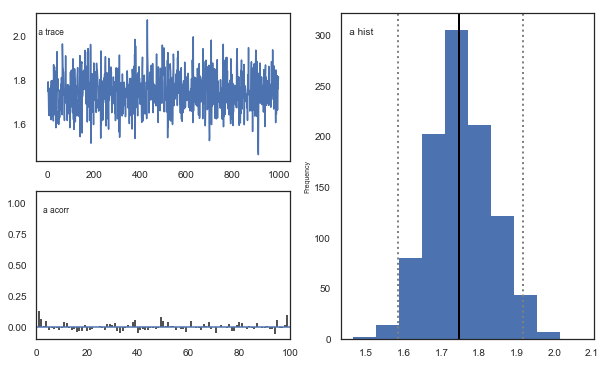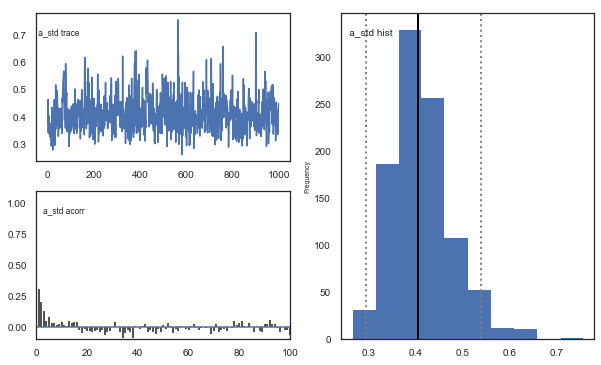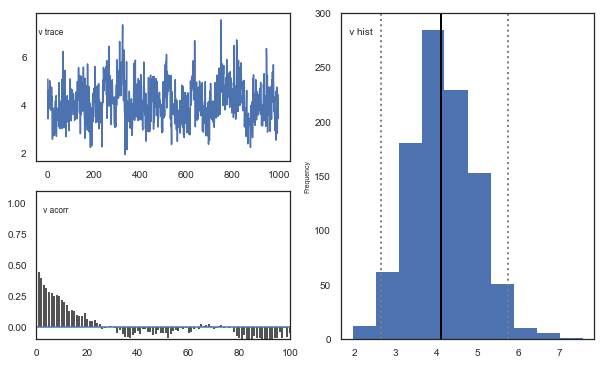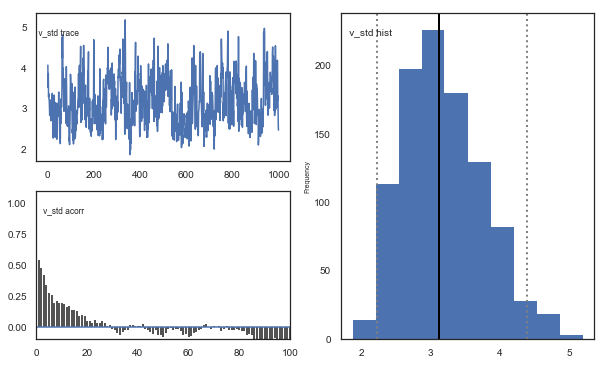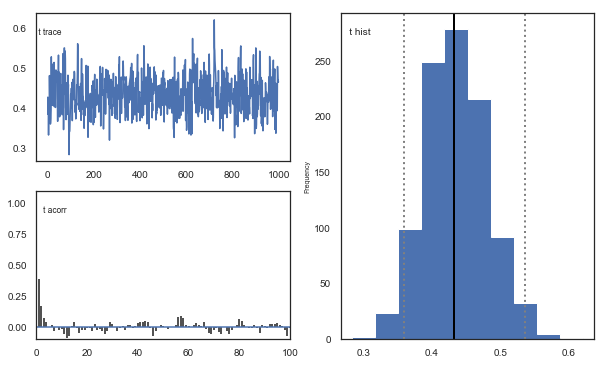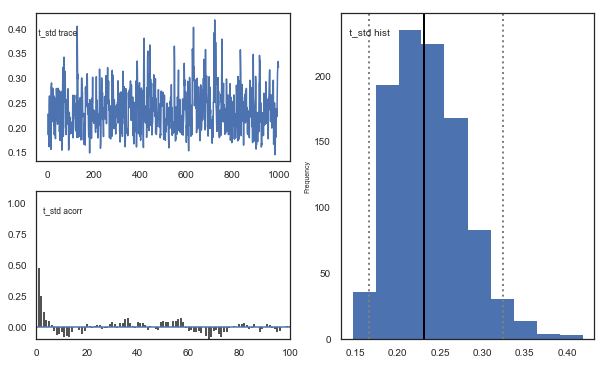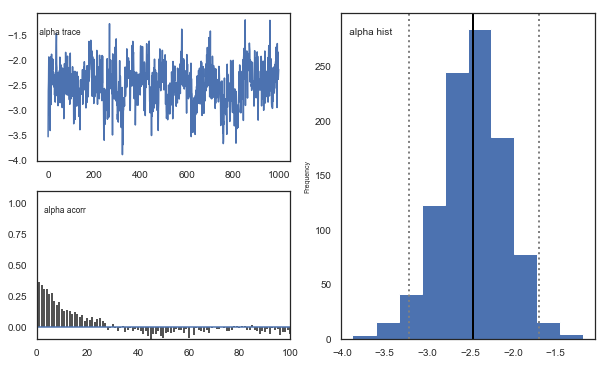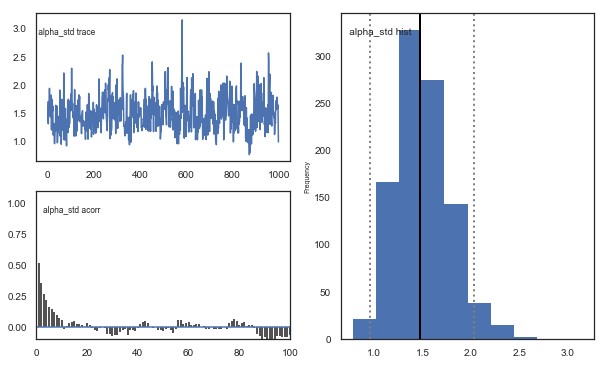Fig. The mixing of the posterior distribution and autocorrelation looks ok.

### Convergence of chains¶

The Gelman-Rubin statistic is a test of whether the chains in the model converges. The Gelman-Ruben statistic measures the degree of variation between and within chains. Values close to 1 indicate convergence and that there is small variation between chains, i.e. that they end up as the same distribution across chains. A common heuristic is to assume convergence if all values are below 1.1. To run this you need to run multiple models, combine them and perform the Gelman-Rubin statistic:

In :
# estimate convergence
from kabuki.analyze import gelman_rubin

models = []
for i in range(3):
m = hddm.HDDMrl(data=data)
m.sample(1500, burn=500,dbname='traces.db',db='pickle')
models.append(m)

gelman_rubin(models)

 [-----------------100%-----------------] 1500 of 1500 complete in 148.3 sec
/Users/madslundpedersen/anaconda/envs/py36/lib/python3.6/site-packages/kabuki/analyze.py:148: FutureWarning:
.loc for label based indexing or
.iloc for positional indexing

See the documentation here:
http://pandas.pydata.org/pandas-docs/stable/user_guide/indexing.html#ix-indexer-is-deprecated
samples[i,:] = model.nodes_db.ix[name, 'node'].trace()
.loc for label based indexing or
.iloc for positional indexing

See the documentation here:
http://pandas.pydata.org/pandas-docs/stable/user_guide/indexing.html#ix-indexer-is-deprecated
return getattr(section, self.name)[new_key]

Out:
{'a': 1.0032460874018214,
'a_std': 1.0009841521872085,
'a_subj.3': 1.0004396186849591,
'a_subj.4': 0.9999534502455131,
'a_subj.5': 1.003486683386713,
'a_subj.6': 1.0019388857243001,
'a_subj.8': 1.0011512646603846,
'a_subj.12': 0.999566242847165,
'a_subj.17': 0.9997315595714608,
'a_subj.18': 1.007802746170568,
'a_subj.19': 1.0006648642694451,
'a_subj.20': 0.9997046168477743,
'a_subj.22': 0.999761021617672,
'a_subj.23': 0.999981103582067,
'a_subj.24': 0.9999604239014099,
'a_subj.26': 0.9999505203703547,
'a_subj.33': 1.0000641985256977,
'a_subj.34': 1.0019030857893532,
'a_subj.35': 0.9995619813519606,
'a_subj.36': 1.0009696684307878,
'a_subj.39': 1.0030366187047326,
'a_subj.42': 1.0004536365990904,
'a_subj.50': 1.0009246525621893,
'a_subj.52': 0.9998106391663311,
'a_subj.56': 1.002833217938155,
'a_subj.59': 1.0037497197540834,
'a_subj.63': 0.9999025733097772,
'a_subj.71': 1.0009484787096814,
'a_subj.75': 1.0130098962514307,
'a_subj.80': 0.9996362431035328,
'v': 1.0205809795803613,
'v_std': 1.017843838450935,
'v_subj.3': 1.0084966266683277,
'v_subj.4': 0.9995268898509083,
'v_subj.5': 1.0055342540841103,
'v_subj.6': 1.006091261867606,
'v_subj.8': 1.0363142858648078,
'v_subj.12': 0.9999768301313733,
'v_subj.17': 1.0071442953265444,
'v_subj.18': 1.0091310053160127,
'v_subj.19': 1.025227632557955,
'v_subj.20': 1.0047516808487722,
'v_subj.22': 0.9995696752962354,
'v_subj.23': 1.0207703297420343,
'v_subj.24': 1.0003032086685848,
'v_subj.26': 1.0063047977790485,
'v_subj.33': 1.007422885046991,
'v_subj.34': 1.0000055617828756,
'v_subj.35': 1.004080679982615,
'v_subj.36': 1.025998215179402,
'v_subj.39': 1.0176678234231622,
'v_subj.42': 1.0446533107136062,
'v_subj.50': 1.0162948205722397,
'v_subj.52': 1.0329846199571966,
'v_subj.56': 1.0187113333213196,
'v_subj.59': 1.0013982327012232,
'v_subj.63': 1.0084242116205158,
'v_subj.71': 1.0034722375822482,
'v_subj.75': 1.0011359012034806,
'v_subj.80': 1.0071658884593422,
't': 1.0005057563118305,
't_std': 1.0022540551935895,
't_subj.3': 1.000335111817265,
't_subj.4': 0.9995572720794353,
't_subj.5': 1.0010911542829217,
't_subj.6': 1.0005078503952383,
't_subj.8': 1.000937902509564,
't_subj.12': 1.0002256652751758,
't_subj.17': 1.0036774720777926,
't_subj.18': 1.0057241781128603,
't_subj.19': 1.000598016225987,
't_subj.20': 1.0000045946218536,
't_subj.22': 0.99983171259197,
't_subj.23': 1.0005420765808288,
't_subj.24': 1.0016080999110242,
't_subj.26': 0.999512841375442,
't_subj.33': 0.9998364286694233,
't_subj.34': 1.0000670998014844,
't_subj.35': 0.9996132338974929,
't_subj.36': 1.0042098480709771,
't_subj.39': 1.0006515809595558,
't_subj.42': 1.000771348791104,
't_subj.50': 1.002215744431939,
't_subj.52': 1.001052279977618,
't_subj.56': 1.0017299690798878,
't_subj.59': 1.0046305114183551,
't_subj.63': 0.9995008742263429,
't_subj.71': 1.001174121937735,
't_subj.75': 1.0153800235690629,
't_subj.80': 0.9999996479882802,
'alpha': 1.0117546794208931,
'alpha_std': 1.016548833490214,
'alpha_subj.3': 1.0095453823767975,
'alpha_subj.4': 0.9999967981599148,
'alpha_subj.5': 1.0013067894285956,
'alpha_subj.6': 1.0015822997094852,
'alpha_subj.8': 1.028833703352052,
'alpha_subj.12': 0.999682056083204,
'alpha_subj.17': 1.002551456868468,
'alpha_subj.18': 1.0128686930345914,
'alpha_subj.19': 1.0216644572624185,
'alpha_subj.20': 1.003469044949529,
'alpha_subj.22': 0.9998485992621597,
'alpha_subj.23': 1.0211627966678443,
'alpha_subj.24': 1.0029351365812929,
'alpha_subj.26': 1.0082171167684313,
'alpha_subj.33': 1.0088110869085494,
'alpha_subj.34': 1.000806268244852,
'alpha_subj.35': 0.9997657478647997,
'alpha_subj.36': 1.016349397314595,
'alpha_subj.39': 1.0154377853905259,
'alpha_subj.42': 1.0380447906771046,
'alpha_subj.50': 1.014307146980194,
'alpha_subj.52': 1.0421339866800452,
'alpha_subj.56': 1.0345554568486672,
'alpha_subj.59': 1.0004809998032806,
'alpha_subj.63': 1.0167696837574038,
'alpha_subj.71': 1.0030045474552403,
'alpha_subj.75': 1.000110789496688,
'alpha_subj.80': 1.0061715847351584}
In :
np.max(list(gelman_rubin(models).values()))

Out:
1.0446533107136062

The model seems to have converged, i.e. the Gelman-Rubin statistic is below 1.1 for all parameters. It is important to always run this test, especially for more complex models (as with separate learning rates for positive and negative prediction errors). So now we can combine these three models to get a better approximation of the posterior distribution.

In :
# Combine the models we ran to test for convergence.
m = kabuki.utils.concat_models(models)


### Joint posterior distribution¶

Another test of the model is to look at collinearity. If the estimation of parameters is very codependent (correlation is strong) it can indicate that their variance trades off, in particular if there is a negative correlation. The following plot shows there is generally low correlation across all combinations of parameters. It does not seem to be the case for this dataset, but common for RLDDM is a negative correlation between learning rate and the scaling factor, similar to what's usually observed between learning rate and inverse temperature for RL models that uses softmax as the choice rule (e.g. Daw, 2011).

In :
alpha, t, a, v = m.nodes_db.node[['alpha', 't', 'a','v']]
samples = {'alpha':alpha.trace(),'t':t.trace(),'a':a.trace(),'v':v.trace()}
samp = pd.DataFrame(data=samples)

def corrfunc(x, y, **kws):
r, _ = stats.pearsonr(x, y)
ax = plt.gca()
ax.annotate("r = {:.2f}".format(r),
xy=(.1, .9), xycoords=ax.transAxes)

g = sns.PairGrid(samp, palette=["red"])
g.map_upper(plt.scatter, s=10)
g.map_diag(sns.distplot, kde=False)
g.map_lower(sns.kdeplot, cmap="Blues_d")
g.map_lower(corrfunc)
g.savefig('matrix_plot.png')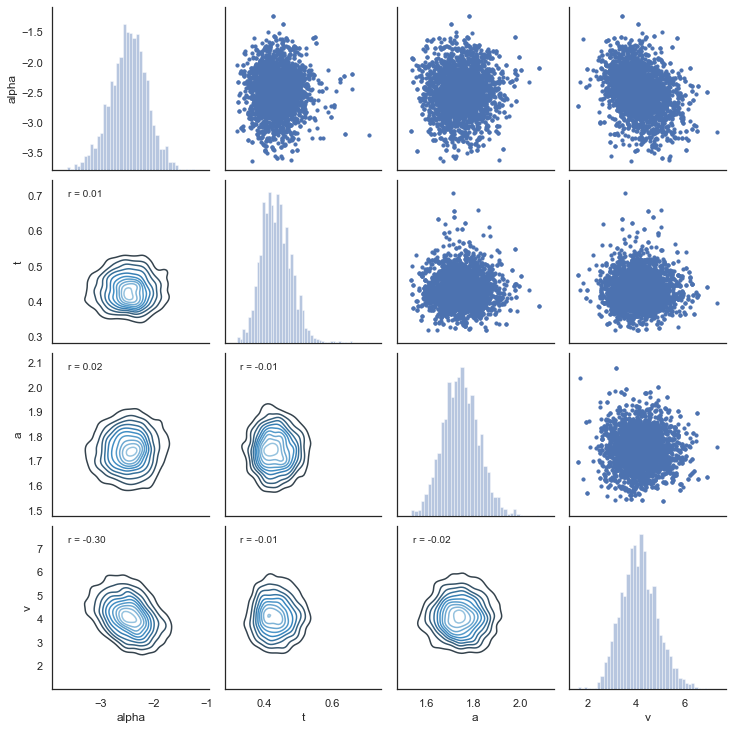## 7. Posterior predictive checks¶

An important test of the model is its ability to recreate the observed data. This can be tested with posterior predictive checks, which involves simulating data using estimated parameters and comparing observed and simulated results.

### extract traces¶

The first step then is to extract the traces from the estimated model. The function get_traces() gives you the samples (row) from the approaximated posterior distribution for all of the estimated group and subject parameters (column).

In :
traces = m.get_traces()

Out:
a a_std a_subj.3 a_subj.4 a_subj.5 a_subj.6 a_subj.8 a_subj.12 a_subj.17 a_subj.18 ... alpha_subj.39 alpha_subj.42 alpha_subj.50 alpha_subj.52 alpha_subj.56 alpha_subj.59 alpha_subj.63 alpha_subj.71 alpha_subj.75 alpha_subj.80
0 1.663258 0.379335 1.999766 1.964781 1.501228 2.558870 2.134354 1.808065 1.428145 1.904139 ... -2.569240 -2.348125 -2.279293 -3.002542 -2.038603 -2.255088 -0.153830 -1.809325 -1.738580 -2.323516
1 1.623170 0.359708 1.912802 1.967012 1.462844 2.466133 2.347265 2.031387 1.476833 1.883079 ... -2.593007 -2.564579 -2.787299 -2.817155 0.128265 -2.358720 -0.709526 -1.876886 -1.428454 -3.140597
2 1.817655 0.312626 2.013651 1.870517 1.438784 2.332917 2.426746 2.079006 1.264283 1.939135 ... -3.187908 -2.566549 -3.341771 -3.206621 -0.724311 -2.446694 -1.133453 -2.153231 -1.589570 -2.702218
3 1.762559 0.573961 1.852805 1.920585 1.456088 2.437470 2.679242 2.099067 1.311264 1.902507 ... -2.045972 -2.466571 -3.093191 -3.204751 -3.220443 -2.381405 -1.060397 -1.521510 -1.892220 -2.902676
4 1.725824 0.472488 1.907957 1.954045 1.462033 2.394734 2.389626 1.928428 1.334218 1.790217 ... -2.035124 -2.679132 -3.821553 -3.372584 -1.139438 -2.372234 -0.895417 -1.900813 -2.196233 -3.063793

5 rows × 120 columns

### simulating data¶

Now that we have the traces the next step is to simulate data using the estimated parameters. The RLDDM includes a function to simulate data. Here's an example of how to use the simulation-function for RLDDM. This example explains how to generate data with binary outcomes. See here for an example on simulating data with normally distributed outcomes. Inputs to function:
a
= decision threshold
t = non-decision time
alpha = learning rate
pos_alpha = defaults to 0. if given it defines the learning rate for positive prediction errors. alpha then becomes the learning rate_ for negative prediction errors.
scaler = the scaling factor that is multiplied with the difference in q-values to calculate trial-by-trial drift rate
p_upper = the probability of reward for the option represented by the upper boundary. The current version thus only works for outcomes that are either 1 or 0
p_lower = the probability of reward for the option represented by the lower boundary.
subjs = number of subjects to simulate data for.
split_by = define the condition which makes it easier to append data from different conditions.
size = number of trials per subject.

In :
hddm.generate.gen_rand_rlddm_data(a=1,t=0.3,alpha=0.2,scaler=2,p_upper=0.8,p_lower=0.2,subjs=1,split_by=0,size=10)

Out:
q_up q_low sim_drift response rt feedback subj_idx split_by trial
0 0.5000 0.50000 0.00000 1.0 0.770 1.0 0 0 1
1 0.6000 0.50000 0.20000 0.0 0.403 0.0 0 0 2
2 0.6000 0.40000 0.40000 0.0 0.612 0.0 0 0 3
3 0.6000 0.32000 0.56000 0.0 0.404 1.0 0 0 4
4 0.6000 0.45600 0.28800 1.0 0.564 1.0 0 0 5
5 0.6800 0.45600 0.44800 1.0 0.416 1.0 0 0 6
6 0.7440 0.45600 0.57600 0.0 0.430 0.0 0 0 7
7 0.7440 0.36480 0.75840 0.0 0.409 0.0 0 0 8
8 0.7440 0.29184 0.90432 1.0 0.361 1.0 0 0 9
9 0.7952 0.29184 1.00672 1.0 0.537 0.0 0 0 10

How to interpret columns in the resulting dataframe
q_up = expected reward for option represented by upper boundary
q_low = expected reward for option represented by lower boundary
sim_drift = the drift rate for each trial calculated as: (q_up-q_low)*scaler
response = simulated choice
rt = simulated response time
feedback = observed feedback for chosen option
subj_idx = subject id (starts at 0)
split_by = condition as integer
trial = current trial (starts at 1)

### Simulate data with estimated parameter values and compare to observed data¶

Now that we know how to extract traces and simulate data we can combine this to create a dataset similar to our observed data. This process is currently not automated but the following is an example code using the dataset we analyzed above.

In :
from tqdm import tqdm #progress tracker
#create empty dataframe to store simulated data
sim_data = pd.DataFrame()
#create a column samp to be used to identify the simulated data sets
data['samp'] = 0
traces = m.get_traces()
#decide how many times to repeat simulation process. repeating this multiple times is generally recommended,
#as it better captures the uncertainty in the posterior distribution, but will also take some time
for i in tqdm(range(1,51)):
#randomly select a row in the traces to use for extracting parameter values
sample = np.random.randint(0,traces.shape-1)
#loop through all subjects in observed data
for s in data.subj_idx.unique():
#get number of trials for each condition.
size0 = len(data[(data['subj_idx']==s) & (data['split_by']==0)].trial.unique())
size1 = len(data[(data['subj_idx']==s) & (data['split_by']==1)].trial.unique())
size2 = len(data[(data['subj_idx']==s) & (data['split_by']==2)].trial.unique())
#set parameter values for simulation
a = traces.loc[sample,'a_subj.'+str(s)]
t = traces.loc[sample,'t_subj.'+str(s)]
scaler = traces.loc[sample,'v_subj.'+str(s)]
alphaInv = traces.loc[sample,'alpha_subj.'+str(s)]
#take inverse logit of estimated alpha
alpha = np.exp(alphaInv)/(1+np.exp(alphaInv))
#simulate data for each condition changing only values of size, p_upper, p_lower and split_by between conditions.
sim_data0 = hddm.generate.gen_rand_rlddm_data(a=a,t=t,scaler=scaler,alpha=alpha,size=size0,p_upper=0.8,p_lower=0.2,split_by=0)
sim_data1 = hddm.generate.gen_rand_rlddm_data(a=a,t=t,scaler=scaler,alpha=alpha,size=size1,p_upper=0.7,p_lower=0.3,split_by=1)
sim_data2 = hddm.generate.gen_rand_rlddm_data(a=a,t=t,scaler=scaler,alpha=alpha,size=size2,p_upper=0.6,p_lower=0.4,split_by=2)
#append the conditions
sim_data0 = sim_data0.append([sim_data1,sim_data2],ignore_index=True)
#assign subj_idx
sim_data0['subj_idx'] = s
#identify that these are simulated data
sim_data0['type'] = 'simulated'
#identify the simulated data
sim_data0['samp'] = i
#append data from each subject
sim_data = sim_data.append(sim_data0,ignore_index=True)
#combine observed and simulated data
ppc_data = data[['subj_idx','response','split_by','rt','trial','feedback','samp']].copy()
ppc_data['type'] = 'observed'
ppc_sdata = sim_data[['subj_idx','response','split_by','rt','trial','feedback','type','samp']].copy()
ppc_data = ppc_data.append(ppc_sdata)
ppc_data.to_csv('ppc_data_tutorial.csv')

100%|██████████| 50/50 [31:09<00:00, 37.39s/it]
/Users/madslundpedersen/anaconda/envs/py36/lib/python3.6/site-packages/pandas/core/frame.py:7138: FutureWarning: Sorting because non-concatenation axis is not aligned. A future version
of pandas will change to not sort by default.

To accept the future behavior, pass 'sort=False'.

To retain the current behavior and silence the warning, pass 'sort=True'.

sort=sort,


### Plotting¶

Now that we have a dataframe with both observed and simulated data we can plot to see whether the simulated data are able to capture observed choice and reaction times. To capture the uncertainty in the simulated data we want to identify how much choice and reaction differs across the simulated data sets. A good measure of this is to calculate the highest posterior density/highest density interval for summary scores of the generated data. Below we calculate highest posterior density with an alpha set to 0.1, which means that we are describing the range of the 90% most likely values.

In :
#for practical reasons we only look at the first 40 trials for each subject in a given condition
plot_ppc_data = ppc_data[ppc_data.trial<41].copy()


### Choice¶

In :
#bin trials to for smoother estimate of response proportion across learning
plot_ppc_data['bin_trial'] = pd.cut(plot_ppc_data.trial,11,labels=np.linspace(0, 10,11)).astype('int64')
#calculate means for each sample
sums = plot_ppc_data.groupby(['bin_trial','split_by','samp','type']).mean().reset_index()
#calculate the overall mean response across samples
ppc_sim = sums.groupby(['bin_trial','split_by','type']).mean().reset_index()
#initiate columns that will have the upper and lower bound of the hpd
ppc_sim['upper_hpd'] = 0
ppc_sim['lower_hpd'] = 0
for i in range(0,ppc_sim.shape):
#calculate the hpd/hdi of the predicted mean responses across bin_trials
hdi = pymc.utils.hpd(sums.response[(sums['bin_trial']==ppc_sim.bin_trial[i]) & (sums['split_by']==ppc_sim.split_by[i]) & (sums['type']==ppc_sim.type[i])],alpha=0.1)
ppc_sim.loc[i,'upper_hpd'] = hdi
ppc_sim.loc[i,'lower_hpd'] = hdi
#calculate error term as the distance from upper bound to mean
ppc_sim['up_err'] = ppc_sim['upper_hpd']-ppc_sim['response']
ppc_sim['low_err'] = ppc_sim['response']-ppc_sim['lower_hpd']
ppc_sim['model'] = 'RLDDM_single_learning'
ppc_sim.to_csv('ppc_choicedata_tutorial.csv')

In :
#plotting evolution of choice proportion for best option across learning for observed and simulated data.
fig, axs = plt.subplots(figsize=(15, 5),nrows=1, ncols=3, sharex=True,sharey=True)
for i in range(0,3):
ax = axs[i]
d = ppc_sim[(ppc_sim.split_by==i) & (ppc_sim.type=='simulated')]
ax.errorbar(d.bin_trial, d.response, yerr=[d.low_err,d.up_err], label='simulated',color='orange')
d = ppc_sim[(ppc_sim.split_by==i) & (ppc_sim.type=='observed')]
ax.plot(d.bin_trial, d.response,linewidth=3,label='observed')
ax.set_title('split_by = %i' %i,fontsize=20)
ax.set_ylabel('mean response')
ax.set_xlabel('trial')
plt.legend()
fig.savefig('PPCchoice.pdf')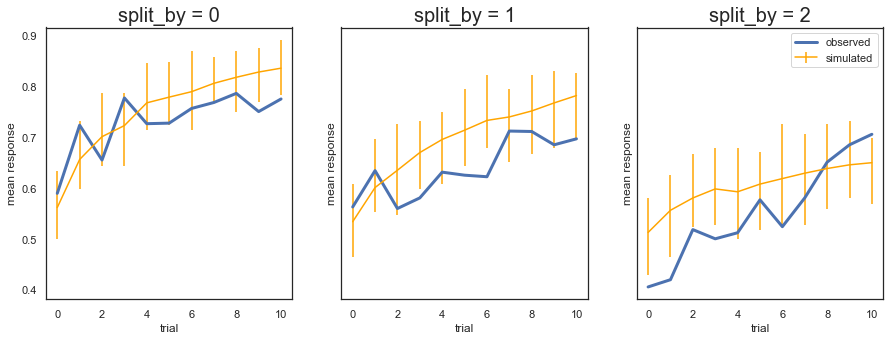Fig. The plots display the rate of choosing the best option (response = 1) across learning and condition. The model generates data (orange) that closely follows the observed behavior (blue), with the exception of overpredicting performance early in the most difficult condition (split_by=2). Uncertainty in the generated data is captured by the 90% highest density interval of the means across simulated datasets.

### RT¶

In :
#set reaction time to be negative for lower bound responses (response=0)
plot_ppc_data['reaction time'] = np.where(plot_ppc_data['response']==1,plot_ppc_data.rt,0-plot_ppc_data.rt)
#plotting evolution of choice proportion for best option across learning for observed and simulated data. We use bins of trials because plotting individual trials would be very noisy.
g = sns.FacetGrid(plot_ppc_data,col='split_by',hue='type')
g.map(sns.kdeplot, 'reaction time',bw=0.05).set_ylabels("Density")
g.savefig('PPCrt_dist.pdf')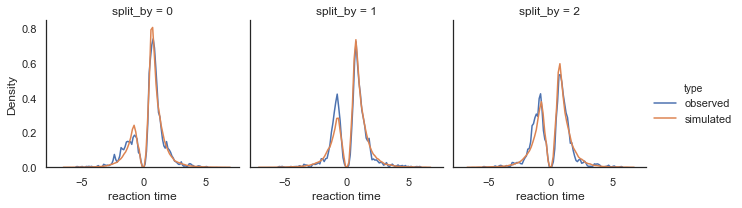Fig. Density plots of observed and predicted reaction time across conditions. RTs for lower boundary choices (i.e. worst option choices) are set to be negative (0-RT) to be able to separate upper and lower bound responses.

## 8. Parameter recovery¶

To validate the RLDDM we ran a parameter recovery study to test to which degree the model can recover the parameter values used to simulate data. To do this we generated 81 synthetic datasets with 50 subjects performing 70 trials each. The 81 datasets were simulated using all combinations of three plausible parameter values for decision threshold, non-decision time, learning rate and the scaling parameter onto drift rate.

### Estimated values split by simulated vales¶

We can plot simulated together with the estimated values to test the models ability to recover parameters, and to see if there are any values that are more difficult to recover than others.

In :
param_recovery = hddm.load_csv('recovery_sim_est_rlddm.csv')

In :
g = sns.catplot(x='a',y='e_a',data=param_recovery,palette='Set1')
g.set_axis_labels("Simulated threshold", "Estimated threshold")
plt.title("Decision threshold")
g.savefig('Threshold_recovery.pdf')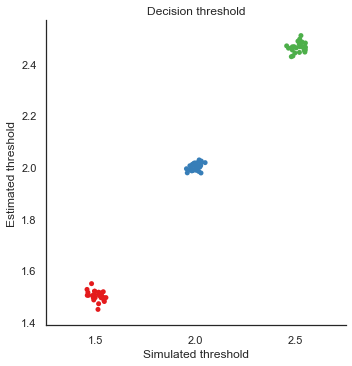In :
g = sns.catplot(x='alpha',y='e_alphaT',data=param_recovery,palette='Set1')
g.set_axis_labels("Simulated alpha", "Estimated alpha")
plt.title("Learning rate")
g.savefig('Alpha_recovery.pdf')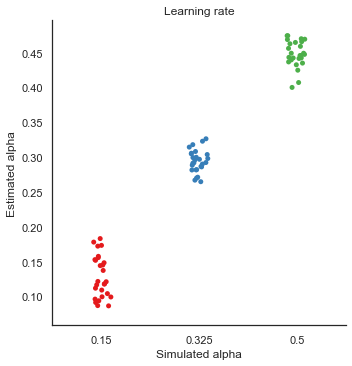In :
g = sns.catplot(x='scaler',y='e_v',data=param_recovery,palette='Set1')
g.set_axis_labels("Simulated scaling", "Estimated scaling")
plt.title("Scaling drift rate")
g.savefig('Scaler_recovery.pdf')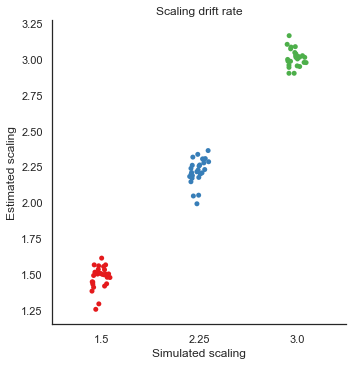In :
g = sns.catplot(x='t',y='e_t',data=param_recovery,palette='Set1')
g.set_axis_labels("Simulated NDT", "Estimated NDT")
plt.title("Non-decision time")
g.savefig('NDT_recovery.pdf')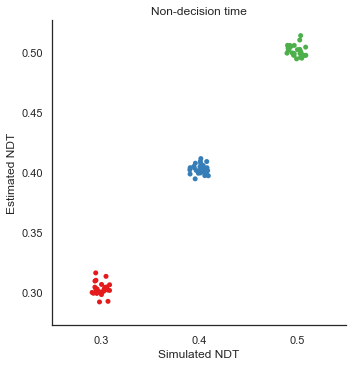Fig. The correlation between simulated and estimated parameter values are high, which means recovery is good. There is somewhat worse recovery for the learning rate and scaling parameter, which makes sense given that they to a degree can explain the same variance (see below).

## 9. Separate learning rates for positive and negative prediction errors¶

Several studies have reported differences in updating of expected rewards following positive and negative prediction errors (e.g. to capture differences between D1 and D2 receptor function). To model asymmetric updating rates for positive and negative prediction errors you can set dual=True in the model. This will produce two estimated learning rates; alpha and pos_alpha, of which alpha then becomes the estimated learning rate for negative prediction errors.

In :
#set dual=True to model separate learning rates for positive and negative prediction errors.
m_dual = hddm.HDDMrl(data,dual=True)
#set sample and burn-in
m_dual.sample(1500,burn=500,dbname='traces.db',db='pickle')
#print stats to get an overview of posterior distribution of estimated parameters
m_dual.print_stats()

 [-----------------100%-----------------] 1500 of 1500 complete in 191.8 sec                        mean         std       2.5q        25q         50q        75q      97.5q       mc err
a                    1.82633   0.0783844    1.67345    1.77512     1.82217    1.87568     1.9965   0.00295453
a_std               0.410321   0.0674141   0.306268   0.364889    0.401635   0.450473   0.564012   0.00270026
a_subj.3             2.17033    0.115262    1.95375    2.09614     2.16539    2.24181     2.4254   0.00548993
a_subj.4             1.89897   0.0579531    1.79347    1.85788     1.89605     1.9393    2.01907   0.00277538
a_subj.5             1.61113    0.082302    1.45691    1.55496      1.6061    1.66577    1.77686   0.00381988
a_subj.6             2.43691    0.101778     2.2429    2.36614     2.43347    2.50934    2.62957   0.00431407
a_subj.8             2.59792    0.144576    2.34397      2.499     2.59463     2.6892    2.92774   0.00726993
a_subj.12            2.06029   0.0799839    1.91855    2.00623     2.05359    2.11537    2.22798   0.00361356
a_subj.17            1.54875    0.100305    1.35735     1.4863     1.54396    1.61257     1.7653    0.0054701
a_subj.18            1.96151    0.122684    1.73789    1.87154     1.96274    2.04099    2.20959   0.00660454
a_subj.19            2.27976    0.108427     2.0839    2.20405     2.27507    2.34357    2.53175   0.00537144
a_subj.20              1.757   0.0906857    1.57908    1.70036     1.75772    1.81488    1.94106   0.00475469
a_subj.22            1.40039   0.0492764     1.3153    1.36434     1.39905    1.43278    1.50517   0.00258208
a_subj.23            1.95365    0.099402    1.77161    1.88705      1.9462    2.01884    2.15432   0.00426745
a_subj.24            1.68369   0.0978159    1.52375    1.61216     1.67733    1.74375    1.90374    0.0047914
a_subj.26            2.23402   0.0798351    2.08258    2.17478     2.23315    2.28916    2.38973   0.00366055
a_subj.33            1.57319   0.0707342    1.44946    1.52259     1.56998    1.62238    1.71148   0.00299887
a_subj.34            1.84701   0.0917249    1.67547    1.78566     1.84312    1.90886    2.02471   0.00440968
a_subj.35            1.85894   0.0913431    1.68528    1.79731     1.86485    1.92094    2.03658   0.00379595
a_subj.36            1.32917   0.0657278    1.20156    1.28636     1.32837    1.36863    1.46548   0.00299776
a_subj.39            1.58224   0.0571074    1.48113    1.54243     1.57864    1.61699    1.70573   0.00272969
a_subj.42            1.85728   0.0910291    1.68796    1.79839     1.85521    1.91323    2.04917   0.00501664
a_subj.50            1.57842   0.0593103    1.47311    1.53575     1.57541    1.61701    1.70146   0.00315943
a_subj.52            2.29593    0.104286    2.10454    2.22466      2.2942    2.35891    2.51119   0.00558897
a_subj.56            1.47579   0.0489699    1.38374    1.44303     1.47525    1.50802    1.57638   0.00203615
a_subj.59            1.41423   0.0838355    1.25798     1.3551     1.41686    1.47376    1.58004   0.00482176
a_subj.63            1.98606    0.105647    1.79733    1.91055     1.97667    2.05667    2.21026   0.00515372
a_subj.71            1.37492   0.0416381    1.29913    1.34635     1.37351    1.40374    1.45383   0.00193188
a_subj.75            1.04381   0.0424396   0.963908    1.01437     1.04343    1.07007     1.1287   0.00215329
a_subj.80            2.20728   0.0949151     2.0232    2.14268     2.20566    2.27035    2.40363   0.00343694
v                    3.66306    0.432881    2.80263    3.36481     3.67691    3.94446    4.53137    0.0179453
v_std                2.16051    0.347665    1.59481    1.92183     2.11674    2.36274    2.98355    0.0183554
v_subj.3             4.05807    0.584568    3.05244     3.6736     4.01923    4.43582    5.33269    0.0211871
v_subj.4             0.93029    0.149903   0.668345   0.825182    0.921209     1.0267    1.25353   0.00749321
v_subj.5             3.10298    0.410738    2.35251    2.81169     3.11047    3.37633    3.94636    0.0155184
v_subj.6             4.37315     1.51163    2.02799    3.22323     4.26505     5.3469    7.84778     0.115543
v_subj.8             2.10131    0.291351    1.55417    1.89635     2.10656     2.2918    2.68403    0.0102131
v_subj.12            3.30997     0.27209    2.79541    3.12027     3.30714    3.51814     3.8123   0.00979875
v_subj.17            4.77054    0.654596    3.61074    4.32052     4.71783    5.15654    6.20269    0.0344306
v_subj.18            5.60507    0.914642    4.05708    4.95358     5.50239    6.14505    7.59222    0.0525223
v_subj.19            6.79667    0.531696    5.84821    6.41609     6.78247    7.15707    7.86691    0.0256374
v_subj.20            5.06997    0.751946    3.80191    4.51495     4.99583    5.54233    6.76405    0.0508111
v_subj.22            7.74229    0.554212    6.66899    7.38473     7.72739    8.10784    8.88024    0.0226088
v_subj.23            1.65386    0.326853    1.05624    1.43946     1.64962    1.84378    2.35648    0.0147053
v_subj.24             4.9282    0.716149    3.58835    4.46113     4.91188    5.38852    6.49608    0.0250187
v_subj.26           0.554038    0.159071   0.317581   0.447227    0.538658   0.634405   0.891184   0.00738979
v_subj.33            1.99289      1.5563 -0.0917214   0.979077     1.61419    2.62817    6.07535    0.0882033
v_subj.34            2.67755    0.743488    1.48379    2.19459     2.61205    3.01255     4.4251    0.0343761
v_subj.35            2.11529    0.418304    1.36401    1.83141     2.11525    2.37647    3.02335    0.0155763
v_subj.36            3.05497     1.16149     1.3637    2.20115     2.92209    3.67182    5.81363    0.0818964
v_subj.39            3.14393     1.01339    1.10825    2.48491     3.15981    3.77087    5.16142    0.0524964
v_subj.42            3.77651    0.368915    3.04478    3.53231     3.76981    4.00682    4.52743    0.0147786
v_subj.50            5.24503    0.428943    4.40896    4.98034     5.23241    5.51769    6.08356    0.0187869
v_subj.52            3.50906    0.403754     2.7295    3.22648     3.50778    3.78182    4.30659    0.0166646
v_subj.56            1.31785    0.983386   0.326642   0.749425     1.12579    1.50799    4.35855    0.0773137
v_subj.59            8.77448     1.21852    6.60213    7.93194     8.66269    9.53013     11.496    0.0836054
v_subj.63            2.23256    0.338367    1.62264    1.98565     2.22861    2.45895    2.91907    0.0129532
v_subj.71            3.79805    0.327746    3.15876    3.57293     3.79705    4.01523    4.43214    0.0119512
v_subj.75            4.97022    0.447116    4.13118    4.65435     4.96064    5.27331    5.81798    0.0167969
v_subj.80            2.12787    0.971202   0.846467    1.41587     1.88945      2.589    4.74006    0.0674847
t                   0.428604    0.044101   0.353065   0.396248    0.425327   0.457601   0.525839   0.00218668
t_std               0.234719   0.0433914   0.164275   0.202987    0.229153   0.262883   0.333006   0.00233875
t_subj.3             1.06301   0.0242696    1.00809    1.04874     1.06433    1.08068     1.1041   0.00106904
t_subj.4            0.528016   0.0181393   0.489347   0.516371    0.529422   0.541093   0.560231   0.00082801
t_subj.5            0.521158   0.0134301   0.491967   0.512937    0.522271   0.530159   0.546061  0.000601849
t_subj.6            0.393535    0.031081   0.332742   0.371696    0.394455   0.414439   0.452232   0.00136618
t_subj.8            0.645487    0.046208   0.548299   0.616548    0.650334   0.677856   0.726427   0.00214894
t_subj.12           0.400791   0.0142303   0.371308   0.392207    0.401693   0.411239   0.427577  0.000644815
t_subj.17            0.49389   0.0160554    0.46639   0.484731    0.493694   0.501411   0.544435  0.000937253
t_subj.18           0.437714    0.024544   0.381513   0.423714     0.44101   0.455774    0.47827   0.00118883
t_subj.19           0.408016   0.0164337   0.370056   0.398919    0.408948   0.419889   0.434781  0.000790638
t_subj.20           0.514031   0.0132655   0.485829   0.505598    0.515052   0.522825   0.537302  0.000720981
t_subj.22           0.325148  0.00616492   0.311362   0.321639     0.32567   0.329167    0.33589  0.000321026
t_subj.23            0.48052   0.0231803   0.427495   0.466163    0.481808   0.497029   0.519889  0.000967584
t_subj.24           0.453918   0.0141035   0.424352   0.444748    0.454901   0.463995   0.478395  0.000737631
t_subj.26           0.443235   0.0347691   0.371609   0.418196    0.448561   0.469806   0.499016   0.00161531
t_subj.33            0.19289   0.0161553   0.163663   0.181761    0.191735   0.203197   0.227826  0.000650216
t_subj.34           0.341855   0.0304285   0.275627   0.325666    0.343243   0.358593   0.411272   0.00135037
t_subj.35           0.323872   0.0241005   0.275647   0.309315    0.323585    0.33817   0.371883  0.000954075
t_subj.36           0.448459   0.0124212   0.418934   0.441661    0.450627   0.457502   0.467191  0.000592505
t_subj.39           0.619463   0.0115061   0.593632   0.612822    0.620391   0.627636   0.639284  0.000519217
t_subj.42            0.38756   0.0160417   0.353556   0.377524    0.388542   0.398015    0.41576  0.000875023
t_subj.50           0.520546   0.0172755   0.482822   0.510046    0.524875   0.533784    0.54486  0.000986671
t_subj.52           0.507414   0.0233708   0.455127   0.493376    0.509698   0.523056   0.550123   0.00114481
t_subj.56           0.116896    0.011136  0.0907044   0.110031    0.117803   0.124486   0.136371  0.000481042
t_subj.59           0.367431   0.0187911    0.33152   0.355044    0.368221   0.377594   0.408335    0.0012207
t_subj.63           0.470553   0.0214161   0.423916   0.457205    0.471826   0.484268   0.508559  0.000923657
t_subj.71           0.156369  0.00617554   0.144548   0.152355    0.156495    0.16055   0.168231  0.000277601
t_subj.75           0.257439  0.00715949   0.240442   0.254217     0.25945   0.262397   0.266872  0.000398967
t_subj.80          0.0402711   0.0164579  0.0108391  0.0281409   0.0399061    0.05107  0.0745975  0.000551567
alpha               -4.78158    0.898295   -6.75036   -5.29889    -4.70286   -4.15113   -3.26662    0.0521409
alpha_std            3.73289     1.06489     2.1336    2.99392     3.52618    4.33781    6.30655    0.0831372
alpha_subj.3        -7.05258     2.50159   -13.1917   -8.32234    -6.52649   -5.18567   -3.96031     0.124696
alpha_subj.4        0.426963     1.11459  -0.944668  -0.230608    0.250964   0.808489    2.92571    0.0541998
alpha_subj.5        -6.91208     2.70265   -14.1073   -8.38504    -6.20221   -4.93885   -3.59952     0.135496
alpha_subj.6         -7.8891      2.5143   -13.9728   -9.12846    -7.44981   -6.13023   -4.37058     0.130676
alpha_subj.8        -2.72998    0.994146   -4.36336   -2.88409    -2.58956   -2.32116   -1.87981    0.0494222
alpha_subj.12       -8.06559     2.69454   -14.9298   -9.48851    -7.54959    -6.1212   -4.43814     0.144476
alpha_subj.17       -6.60254     2.96442   -14.1679   -7.99399     -5.9254   -4.45374   -2.86398     0.158913
alpha_subj.18       -6.07482     2.68135   -13.1222   -7.13457    -5.22561   -4.18235   -3.13356     0.136542
alpha_subj.19       -3.92613    0.285418   -4.64184   -4.07693    -3.89082   -3.72418   -3.45543    0.0101292
alpha_subj.20       -3.50676    0.516536   -4.68548   -3.79117    -3.49135   -3.17623   -2.56029    0.0285649
alpha_subj.22        -9.2371     2.06096    -13.833   -10.3452    -8.81722   -7.74703   -6.32702    0.0931406
alpha_subj.23       -4.31396     2.64539   -11.1804   -5.27713    -3.51936   -2.54338   -1.35995     0.136443
alpha_subj.24       -1.55403    0.339719   -2.20419   -1.76759      -1.541   -1.34377  -0.857031    0.0101265
alpha_subj.26         2.3789     2.61685   -0.93534    1.21823     2.23266    3.52459    7.21916     0.174057
alpha_subj.33         -5.213      4.0036   -13.9326   -7.62198    -5.00017   -2.53649    1.71173     0.184676
alpha_subj.34       -5.53051     2.76871   -12.5893   -6.87964    -4.73883   -3.58031   -2.30041     0.141782
alpha_subj.35       -6.38576     3.13276   -14.0043    -7.9697    -5.83136    -4.1429   -2.09597     0.146654
alpha_subj.36       -1.79782     2.08031   -8.22385   -2.23937    -1.26025   -0.71467   0.467845     0.114779
alpha_subj.39       -5.44883     3.89269   -13.4074   -7.56688    -5.38491    -3.9298    2.91726     0.306435
alpha_subj.42       -5.04472     2.33085   -11.3301   -5.17445    -4.30883    -3.8231   -3.27149     0.132284
alpha_subj.50       -8.08276     2.46464    -14.181   -9.24783    -7.53744   -6.25954     -4.971     0.126942
alpha_subj.52       -3.82568    0.752882   -5.04399   -4.00424    -3.71483   -3.47445   -3.12819    0.0371159
alpha_subj.56       -5.44862     3.41322   -13.5386   -7.36032    -4.89808   -3.09328   -0.30246      0.18234
alpha_subj.59       -3.44337    0.488828   -4.45293   -3.66276    -3.39263   -3.14521   -2.72879    0.0247054
alpha_subj.63       -6.90635     2.82143   -13.3582   -8.47125    -6.38258   -4.78577   -3.09205     0.119088
alpha_subj.71       -8.45649     2.63845   -15.2664   -9.69164    -7.92428   -6.56692   -5.18337     0.138627
alpha_subj.75       -7.48641     2.73537   -14.0637   -8.70384    -6.88744   -5.49349   -3.90752     0.134957
alpha_subj.80       -5.58797     3.14239    -13.527   -7.20775    -4.67266   -3.26561   -1.78386     0.173523
pos_alpha          -0.895935    0.339247   -1.54324   -1.11526   -0.909311  -0.674954  -0.160331    0.0180688
pos_alpha_std        1.45037    0.313221   0.953754    1.23314     1.42053     1.6352    2.12882    0.0199312
pos_alpha_subj.3   -0.739699    0.608631   -1.89483   -1.10114   -0.748097  -0.389608   0.549614    0.0252056
pos_alpha_subj.4    0.501533    0.880432  -0.924143  -0.107416    0.414538    1.00973    2.65335    0.0414756
pos_alpha_subj.5    0.343363       0.823  -0.927268  -0.207032     0.20958   0.824743    2.30273    0.0313956
pos_alpha_subj.6    -3.57442    0.488523   -4.37085   -3.91692    -3.64022   -3.26027   -2.50903    0.0374053
pos_alpha_subj.8    0.449901    0.783986  -0.861785 -0.0643286    0.390448   0.894634    2.34131    0.0299916
pos_alpha_subj.12   0.402094     0.39053  -0.288765   0.152244    0.383246   0.612424    1.26959    0.0123956
pos_alpha_subj.17   -1.03625    0.728279   -2.28107   -1.47547    -1.09125  -0.741944   0.869704    0.0404402
pos_alpha_subj.18   -1.95641    0.360458   -2.68864   -2.18083    -1.95473   -1.71167   -1.28532     0.016443
pos_alpha_subj.19   -1.67176    0.221585   -2.15427   -1.80486    -1.65991   -1.51665   -1.28461   0.00811834
pos_alpha_subj.20    -2.1366    0.329426   -2.81754   -2.35952    -2.11302   -1.90687   -1.52181    0.0211777
pos_alpha_subj.22   -1.52007    0.125742   -1.78746   -1.59736    -1.51762   -1.43222   -1.28096   0.00531203
pos_alpha_subj.23  0.0529679     1.00904   -1.98607  -0.507643  0.00876946   0.620401    2.14044    0.0414709
pos_alpha_subj.24   -1.34182    0.309116   -1.93674   -1.55106    -1.33801   -1.14381   -0.73865    0.0112161
pos_alpha_subj.26  0.0893943    0.970026   -1.58794  -0.484993    -0.01053   0.619843    2.44391    0.0521637
pos_alpha_subj.33   -2.24994      1.2274   -4.38351   -3.11987    -2.30015   -1.48697   0.414115    0.0705896
pos_alpha_subj.34  -0.704432     1.05906   -2.88173   -1.33173   -0.550406  0.0335074    1.12025    0.0505609
pos_alpha_subj.35  -0.739327    0.629917   -2.36813   -1.05908   -0.672616  -0.324667   0.371349    0.0253342
pos_alpha_subj.36   -1.56092      1.5089   -3.96919   -2.71246      -1.819   -0.56486    1.61682     0.107652
pos_alpha_subj.39   0.185904     1.77976   -4.36138  -0.191296    0.508164    1.23217      2.711     0.155413
pos_alpha_subj.42  -0.603407    0.253323   -1.11295  -0.760958   -0.598121  -0.435014  -0.103201   0.00879472
pos_alpha_subj.50   -1.37377    0.262776   -1.90291    -1.5452    -1.37773   -1.20679  -0.872482   0.00894318
pos_alpha_subj.52  0.0772404     0.36944  -0.635686  -0.178077   0.0697795   0.304712   0.851372     0.011965
pos_alpha_subj.56   -1.51497     1.97144   -5.41133   -2.83118    -1.37977   -0.24753    2.36421     0.128189
pos_alpha_subj.59   -2.10815    0.279822   -2.66591    -2.2839    -2.11047   -1.92215   -1.56226    0.0176411
pos_alpha_subj.63    1.29028    0.873231  -0.164205    0.68542     1.18272     1.8369    3.14479    0.0375058
pos_alpha_subj.71   -1.70335     0.21584    -2.1278   -1.83928    -1.70867   -1.56798   -1.24251   0.00688448
pos_alpha_subj.75  -0.502723    0.297922   -1.07137  -0.704546   -0.508679  -0.304064  0.0950879    0.0107456
pos_alpha_subj.80   -1.61934     1.26102   -3.49573   -2.52006    -1.82877  -0.950009    1.36519    0.0865199
DIC: 9186.303547
deviance: 9083.079636
pD: 103.223911

In :
m_dual.plot_posteriors()

Plotting a
Plotting a_std
Plotting v
Plotting v_std
Plotting t
Plotting t_std
Plotting alpha
Plotting alpha_std
Plotting pos_alpha
Plotting pos_alpha_std